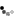Loading... Please wait...

• My Account
• Order Status

# Converting Vibration Amplitude Readings to Unbalance Tolerances

Posted

www.balancingweights.com

CONVERTING AMPLITUDE READINGS (MILS, IN/SEC, G’S) TO UNBALANCE TOLERANCES (OZ-IN, GRAM-IN, GR-MM)

There are times when it is necessary to convert amplitude readings such as Mils or Velocity to balance terms of ounce inches or gram inches. A detailed method will be outlined in next weeks article, CHECKING OR PROVING THE BALANCE LEVELS IN ROTORS. There are times, however, when just a quick check is required for informational purposes, this paper addresses that.

There are times when it is desirable to convert vibration amplitude readings to unbalance tolerance terms. An example would be when the balancing machine being used does not read out in ounces or grams, however, there are many times when a faster, less precise method will serve the purpose.The following discussion will outline several ways this can be accomplished for different applications.The examples shown are for single plane, single weight corrections.The steps should be repeated for standard two plane balancing.

CASE 1

You are requested to balance a symmetrical, 1000 pound rotor to 2.0 ounce inches per plane and 2 plane correction is required.The instrumentation available to balance reads only in mils of amplitude.What approximate mils level would be required ?

PROCEDURE

STEP 1.Spin the rotor and record the original vibration reading and the phase angle.Vibration Reading = ________Phase Angle = _________

STEP 2.Add a Calibration Weight to the rotor in the plane being checked at

any convenient location.

Calibration Weight = ______ oz. Radius it was added = ________ in.

STEP 3.Multiply Weight x Radius to calculate ounce inches = ________ oz-in.

STEP 4.Spin the rotor and record the new level of vibration and phase.

Vibration Reading = _________ Phase Angle = __________

STEP 5.Draw the Vector Diagram (shown below)

STEP 6.Connect the ends of the two vectors from Step 1 and Step 4

.

STEP 7.Measure the length of the connecting vector and record.

Vector length = _______ mils.

STEP 8.Calculate the mils reading required from the following formula:

Mils Required = (Step 7 Vector length)x (requested tolerance)

(Step 3 value)

EXAMPLE

Requested tolerance = 2 oz-in per plane

Original Vibration Reading (A on Vector Plot) = 3. 0 mils at 0 degrees.

Ounce inches = 5 x 10 = 50

= 4.0 mils at 90 degrees

Measure the length of C on Vector Plot = 5 mils

Mils reading to meet tolerance = (5 mils)x(2 oz-in) /(50 oz-in) = 0.2 mils

There are other methods of calculating this tolerance without vectors if the proper tools are available.Any instrument, calculator, or computer program that contains the solution for a single plane balance can be used in place of drawing the vectors by following these Steps.Typical Vector Diagram

CASE 2

A rotor has been balanced to a mils level deemed acceptable and now the rotor needs to be checked to determine the ounce inch tolerance achieved.As mentioned earlier, Balance Tip 101 is the standard procedure for checking unbalance levels, however the following procedure can be used for a good approximation.

Follow the same Steps as previously outlined.

Step 1.Spin the rotor and record final vibration amount and phase reading.

Step 2.Add a calibration weight to the rotor in the properplane.

Step 3.Calculate the total oz-in for weight (Weight x Radius)

Step 4.Spin the rotor and record the new vibration amount and phase reading.

Step 5.Draw the Vector Diagram.

Step 6.Connect the end of the two vectors (Steps 1 and 4).

Step 7.Measure the length of the connecting vector.

Step 8.Calculate the remaining unbalance in this plane of the rotor

using the formula;

Unbalance level (oz-in) = (Step 3 ounce inches) x (Step 1 Mils)

(Step 7, Mils)

EXAMPLE

Final Vibration Reading (A on Vector Plot) = 0.3 mils at 0 degrees

Test Weight = 1 ounceRadius = 10 inches,

Test Weight = 10 oz - in.

Vibration Reading with Test Weight (B on Vector Plot)= 0.4 mils at 90 degrees

Length of (C) on Vector Plot = 0.5 mils

Remaining unbalance in plane = 10 oz-in x 0.3 mils / 0.5 mils = 6 oz-in

As was the mentioned in Case 1, there are other ways to calculate the remaining unbalance in a plane of a rotor.Any instrument, calculator or computer program that contains the solution for single plane balance can be used by following the Steps outlined in this Balancing Tip.

It needs to be pointed out again that CHECKING OR PROVING THE BALANCE LEVELS IN ROTORS covers the accepted method of proving rotor balance.The procedures in this Tip are for convenience checks. Also, the checks need to be made for both planes in two plane balancing applications.

Although C D International, Inc believes reasonable efforts have been made to ensure the accuracy of the information contained in this document, it may include inaccuracies or typographical errors and may be changed or updated without notice. It is intended for discussion and educational purposes only and is provided "as is" without warranty of any kind and reliance on any information presented is at your own risk.